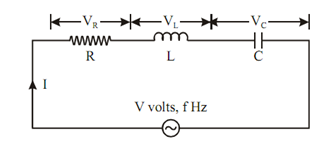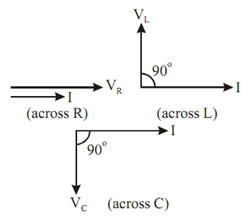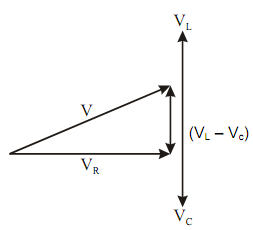## Series and Parallel Resonance Assignment Help

Assignment Help: >> AC Circuits - Series and Parallel Resonance

Series and Parallel Resonance:

Resonance is that condition in any AC circuit at a specific frequency, while the applied voltage and resultant current are in same phase. Thus, at resonance the power factor of the AC circuit is unity and it acts like a pure resistive circuit.

Series Resonance

In series RLC circuit at a specific frequency when inductive reactance (XL = ωL) becomes equal to the capacitive reactance ( X C =   1/ ωC  ), then the circuit is said to be at resonance

XL = XC

In the series RLC circuit of Figure, the circuit current I is specified byFigure: Series Resonance Circuit

I =( V/ Z)  A

where Z represents the equivalent impedance of the circuit.

Z = R + j ωL +(1/ j ωC)

= R + j ωL -(1/ ωC)j

Z = R + j ( X L  - X C )

where

X L        = ωL and  XC    =   1/ ωC

= R +  jX

where (XL - XC) = X (Net Reactance)

Therefore

I =        V / (R + j ( X L - X C ))  = V / R +  jX    AmpFigure: Voltage and Current Phasor

And voltage drop across resistance, R is VR = IR (in phase with I)

Voltage drop across inductance, L is VL = I XL (leading I by 90o)

Voltage drop across capacitance, C is VC = I XC (leading I by 90o)

The phasor diagram of the variables of the entire circuit is shown in FigureFigure: Voltage Vector Diagram of RLC Series Circuit

 Admittance Curve Band Width Impedance Curve Parallel Resonance Voltage Vector Diagram#### Assured A++ Grade

Get guaranteed satisfaction & time on delivery in every assignment order you paid with us! We ensure premium quality solution document along with free turntin report!

All rights reserved! Copyrights ©2019-2020 ExpertsMind IT Educational Pvt Ltd